Search ResultsCollection Resources(7)

View
Selected filters:
• Angular Velocity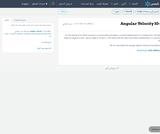Conditions of Use:
Remix and Share
Rating

Where R is the radius of the circle where the object rotates.

Subject:
Applied Science
Material Type:
Syllabus
Provider:
King Abdulaziz University
Author:
سيد حجازي
Date created
4-صفر-1440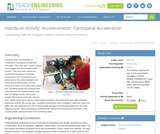Conditions of Use:
Rating

Students work as physicists to understand centripetal acceleration concepts. They also learn ...

Students work as physicists to understand centripetal acceleration concepts. They also learn about a good robot design and the accelerometer sensor. They also learn about the relationship between centripetal acceleration and centripetal force governed by the radius between the motor and accelerometer and the amount of mass at the end of the robot's arm. Students graph and analyze data collected from an accelerometer, and learn to design robots with proper weight distribution across the robot for their robotic arms. Upon using a data logging program, they view their own data collected during the activity. By activity end , students understand how a change in radius or mass can affect the data obtained from the accelerometer through the plots generated from the data logging program. More specifically, students learn about the accuracy and precision of the accelerometer measurements from numerous trials.

Subject:
Engineering
Physics
Material Type:
Activity/Lab
Provider:
TeachEngineering
Provider Set:
TeachEngineering NGSS Aligned Resources
Author:
AMPS GK-12 Program,
Carlo Yuvienco
Jennifer S. Haghpanah
Date created
14-محرم-1436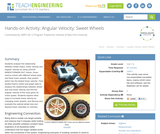Conditions of Use:
Rating

Students analyze the relationship between wheel radius, linear velocity and angular velocity ...

Students analyze the relationship between wheel radius, linear velocity and angular velocity by using LEGO(TM) MINDSTORMS(TM) NXT robots. Given various robots with different wheel sizes and fixed motor speeds, they predict which has the fastest linear velocity. Then student teams collect and graph data to analyze the relationships between wheel size and linear velocity and find the angular velocity of the robot given its motor speed. Students explore other ways to increase linear velocity by changing motor speeds, and discuss and evaluate the optimal wheel size and desired linear velocities on vehicles.

Subject:
Engineering
Material Type:
Activity/Lab
Provider:
TeachEngineering
Provider Set:
TeachEngineering NGSS Aligned Resources
Author:
AMPS GK-12 Program, Polytechnic Institute of New York University,
James Muldoon, Kelly Brandon, Jigar Jadav
Date created
4-محرم-1437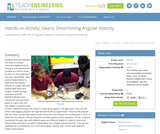Conditions of Use:
Rating

Students work as engineers and learn to conduct controlled experiments by changing ...

Students work as engineers and learn to conduct controlled experiments by changing one experimental variable at a time to study its effect on the experiment outcome. Specifically, they conduct experiments to determine the angular velocity for a gear train with varying gear ratios and lengths. Student groups assemble LEGO MINDSTORMS(TM) NXT robots with variously sized gears in a gear train and then design programs using the NXT software to cause the motor to rotate all the gears in the gear train. They use the LEGO data logging program and light sensors to set up experiments. They run the program with the motor and the light sensor at the same time and analyze the resulting plot in order to determine the angular velocity using the provided physics-based equations. Finally, students manipulate the gear train with different gears and different lengths in order to analyze all these factors and figure out which manipulation has a higher angular velocity. They use the equations for circumference of a circle and angular velocity; and convert units between radians and degrees.

Subject:
Engineering
Material Type:
Activity/Lab
Provider:
TeachEngineering
Provider Set:
TeachEngineering NGSS Aligned Resources
Author:
AMPS GK-12 Program,
Jennifer S. Haghpanah, Leonarda Huertas, Jasmin Mejias, Mihai Pruna, James Cox
Date created
14-محرم-1436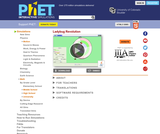Conditions of Use:
Rating

Join the ladybug in an exploration of rotational motion. Rotate the merry-go-round ...

Join the ladybug in an exploration of rotational motion. Rotate the merry-go-round to change its angle, or choose a constant angular velocity or angular acceleration. Explore how circular motion relates to the bug's x,y position, velocity, and acceleration using vectors or graphs.

Subject:
Physics
Material Type:
Simulation
Provider:
Provider Set:
PhET Interactive Simulations
Author:
Gratny, Mindy
Kathy Perkins
Mindy Gratny
Perkins, Kathy
PhET Interactive Simulations
Reid, Sam
Sam Reid
Date created
2-ذو القعدة-1432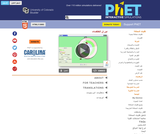Conditions of Use:
Rating

Join the ladybug in an exploration of rotational motion. Rotate the merry-go-round ...

Join the ladybug in an exploration of rotational motion. Rotate the merry-go-round to change its angle, or choose a constant angular velocity or angular acceleration. Explore how circular motion relates to the bug's x,y position, velocity, and acceleration using vectors or graphs.

Subject:
Physics
Material Type:
Simulation
Provider:
Provider Set:
PhET Interactive Simulations
Author:
Gratny, Mindy
Perkins, Kathy
Reid, Sam
Date created
17-ذو القعدة-1434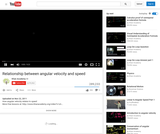Conditions of Use:
Remix and Share
Rating

This 9-minute video lesson looks at how angular velocity relates to speed.

Subject:
Physics
Material Type:
Lecture
Provider: